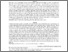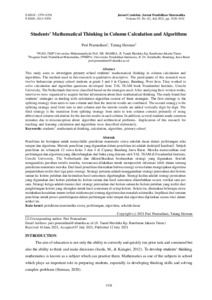# Students' Mathematical Thinking in Column Calculation and Algorithms

Pramudiani, Puri dan Herman, Tatang (2021) Students' Mathematical Thinking in Column Calculation and Algorithms. Jurnal Cendekia : Jurnal Pendidikan Matematika, 5 (2). pp. 1928-1942. ISSN 2579-9258Preview Text 01_A_2021_Article_Cendekia_Puri_Prof Tatang_Column Calculation.pdf Download (955kB) | Preview

## Abstract

This study aims to investigate primary school students' mathematical thinking in column calculation and algorithms. The method used in this research is qualitative descriptive. The participants of this research were twelve Indonesian primary school students at grade 3 and 4 in Ciparay, Bandung, West Java. They worked to solve calculation and algorithm questions developed from TAL TEAM book Freudenthal Institute, Utrecht University, The Netherlands that were classified based on the strategies used. After analyzing their written works, interviews were organized to acquire further information about their mathematical thinking. The study found that students' strategies in dealing with calculation algorithm consist of three strategies. The first strategy is the splitting strategy from units to tens column and then the interim results are combined. The second strategy is the splitting strategy used from tens to unit column and the interim results are added vertically digit-by-digit. The third strategy is the transition from splitting strategy from units to tens column consists primarily of using abbreviated column calculation for the interim results in each column. In addition, several students made common mistakes due to misconception about algorithm and arithmetical problems. Implication of this research for teaching and learning calculation and algorithms were described elaborately.

Item Type: Article Z Bibliography. Library Science. Information Resources > ZA Information resources > ZA4450 Databases Fakultas Keguruan & Ilmu Pendidikan > Pendidikan Guru Sekolah Dasar Puri Pramudiani 09 Feb 2022 04:27 09 Feb 2022 04:27 http://repository.uhamka.ac.id/id/eprint/12689View Item